# Maze Path-finding using DFS

Ever wondered how to solve a maze effectively?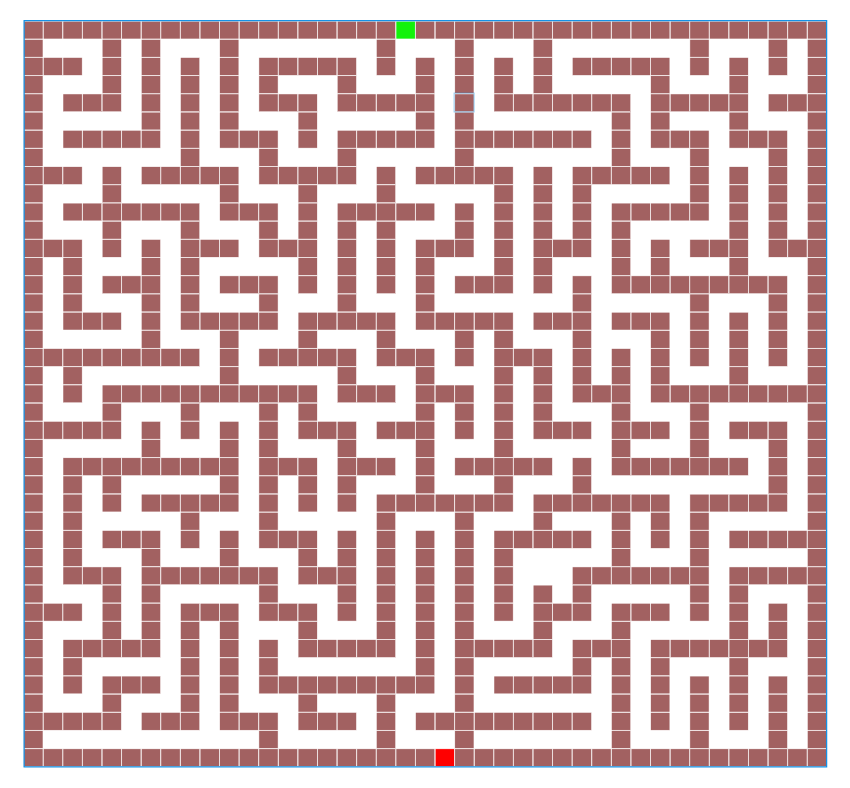41*41 Maze will be solved effectively using DFS Algorithm

Above is the given maze that we will be solving using DFS algorithm. The Green block and the Red block are the starting and ending point respectively.

Depth-first search (DFS) is an algorithm for traversing or searching tree or graph data structures.
In simple words using some traversing technique, we can visit all the nodes of a graph in a certain order that we define.

Of course we will be using Iteration because lets face it, we all hate Recursion (technically I don’t understand recursion 😥😥).

• Vector — to represent a maze in 2D format.
• Stackto store pair of indices of the actual traversal path.
• HashMap — In C++ it is known as unordered_map. This will keep track of all the pair of indices that we have visited.
• Pair — to store the pair of indices. In C++ we use pair<int, int>
• Firstly we will define a 2D vector to represent a maze.
Below is representation of a maze in 2D vector format.
‘X’ => barrier
‘O’ => empty space
‘S’ => start point
‘E’ => end point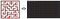Representation Maze in 2D vector format
• Below is the pseudocode for Depth-first search function that will give us the final path to follow.
`def Depth_First_Search(){    pair start_index = find_start_index(maze);    pair current_index = start_index;    stack path_stack;    path_stack.push(start_index);    isVisited[start_index] = true;    while(maze[current_index] != 'E')    {        if(isNextMovePossible(current_index))        {            current_index = nextFesableMove(current_index);            isVisited[current_index] = true;            path_stack.push(current_index);        }        else        {            path_stack.pop();            current_index = path_stack.top();        }    }    return path;}`
• find_start_index(maze) = This function will find ‘S’ or the starting index using for loops.
• isNextMovePossible(current_index) = From a given index, this will check in a particular order if the next move is possible.
For eg:- In below picture our current_index(yellow circle) is at (3,5). From this point, following the given visiting order, we will have to visit Up(because clearly we cannot visit Down or Right). So our current_index will be updated to (2,5).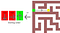Node Visiting order we are following for DFS

Note: If isNextMovePossible(current_index) returns false, that means none of the visiting order is possible, this means we must have taken the wrong step. So we backtrack i.e., remove the current_node from path_stack and set current_node to path_stack’s top and check isNextMovePossible(current_node).
And this process is repeated.
Take a look at the below picture to understand. Our current_index is stuck at an index(yellow circle). From there it backtracks to previous index. Again stuck, again backtrack…and so on..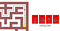Backtracking 4 times since no next Move is possible
• nextFesableMove(current_index) = This utility function will simply update the current_index with the next possible move according to the visiting order.

And Voila……….. We are done. After following the above steps we will get the solution to the Big Maze we started with —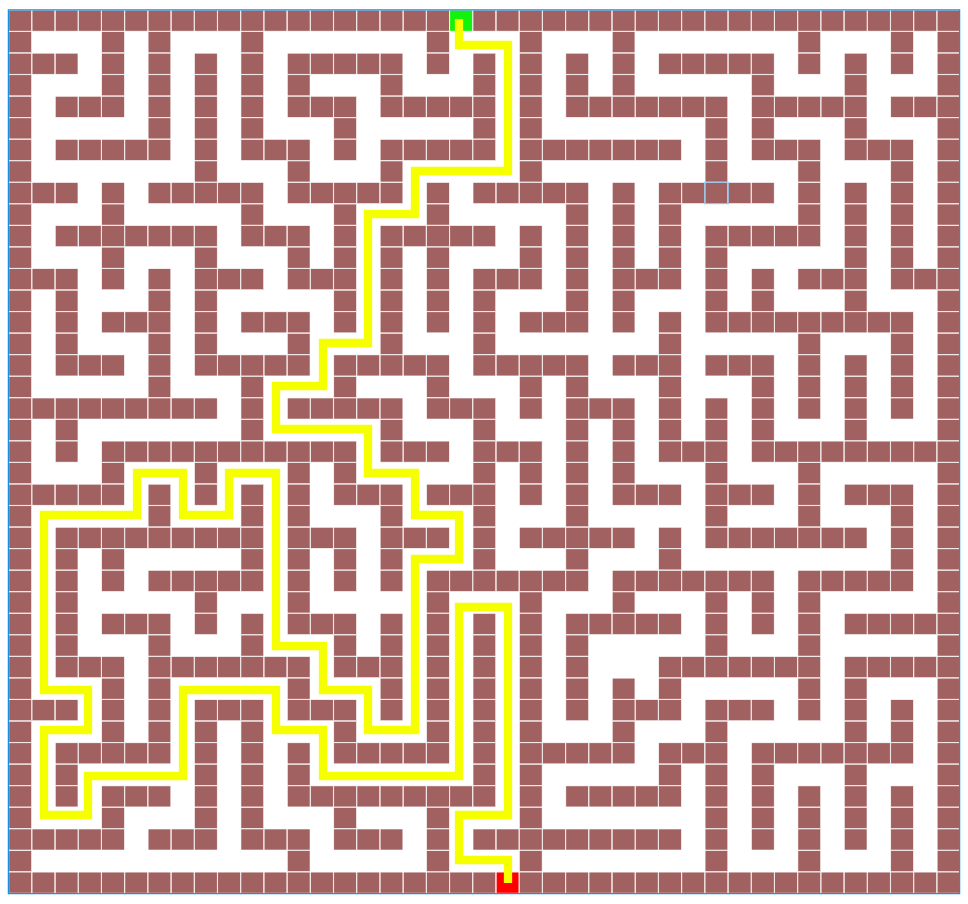Final Solution of the Maze we started with

Also checkout the full code using C++ on my github account.

Thank You for reading so far. Its my first post on Medium… 😊